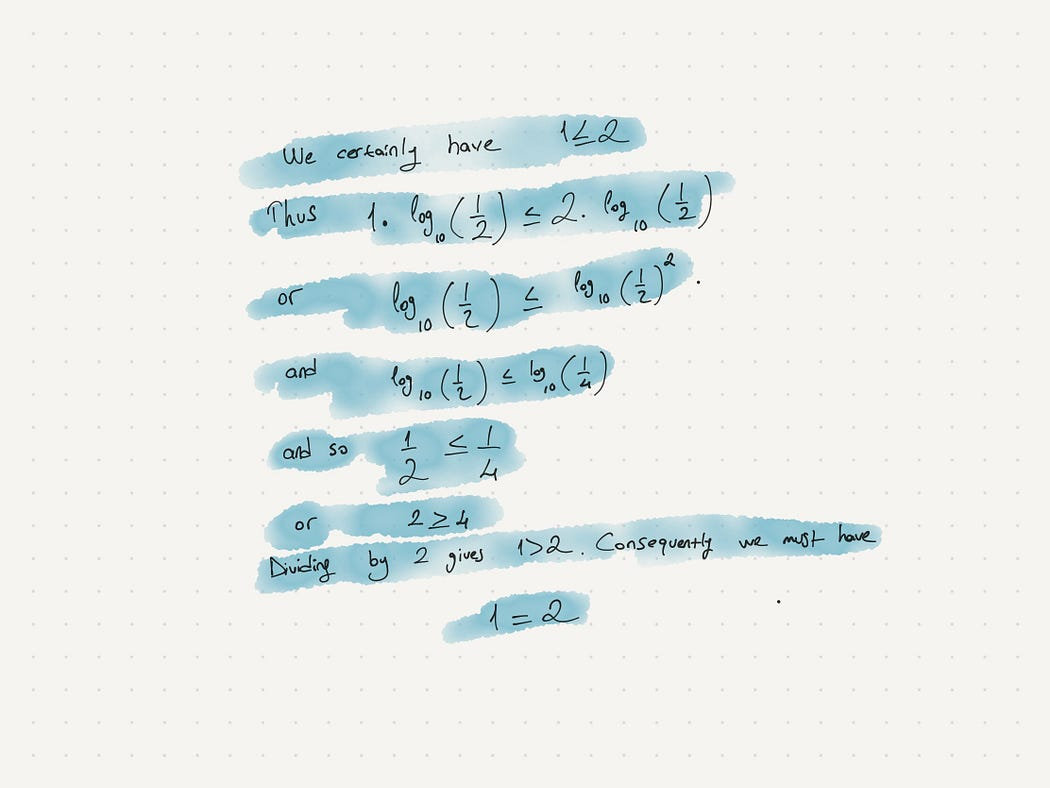# Maths

One plus one, does it make one?

# Maths

##### One plus one, does it make one?

These equations are classics of mathematics or a mathematical fallacy which lead us to absurd results. It may sound ridiculous, but I will prove it to you. Please keep suspecting the proof.In the past, there was a friend of Galileo, called Del Monte. He was believing creation of something out of nothing like Archimedes. When he first proved that 0=1, he had established the existence of God. For him ; God was 1 and 0 was nothing. Thus, God was always exist. Okay, how did he prove that 0=1? Or 1=2? There are certainly a number of convincing ways to prove. And when we collect them together they produce a very convincing document possibly leading the reader to believe these absurdities. But do not worry, we will also see the fault with each proof lie. If 1=2, you and me have to be the same person. But you are you, I am me. It is a logical deduction. problem unless we understand the chemical mechanism behind it.’

Proof of “0=1” Let “a” and “b” each equal to 1.
Since a and b are equal to 1;
b² = ab
Since a equals itself, it is obvious that
a²=a²
Now, let’s try to subtract b² and ab from a². This move yields
a²- b² = a²- ab.
Beautiful.
As you can see, we should try to find the factors of the equations.
a² – ab = a•(a-b).
Likewise, a²-b² = (a+b)•(a-b).Friends, amigos, Romans!!! We are just doing basic Algebra. This statements are perfectly true.
(a+b)•(a-b)=a•(a-b)
So far, so good. Now we need to do two more Algebraic moves. First, divide both sides of the equation by (a-b) and we get
a+b=a
Secondly, subtract a from both sides and we get
b=0
But, wait a minute. I thought we said b is equal to 1 at the very beginning of this proof, so this means that
1=0…
This is probably the most important result we have ever seen so far. Now you can take this result with you and go further. For instance, Einstein had a powerful brain. But wait again, we got 1=0. So that means that Einstein had no brain!!!
Obviously, there is something wrong with our proof… We made a mistake.
Do you remember that we divide our equations by “a - b”. But look out! “a” and “b” are both equal to 1 and that’s why “a — b” is going to equal to “0.” We divided something by zero.

We destroyed the world. Don’t forget that. You can destroy the logic with zero! If you are wondering why we can not divide by 0. You can read my previous article. Here some additional proofs.Yafang Cheng from the Max-Planck Institute for Chemistry in Germany believes that the discovery will help to develop more locally tailored strategies. ‘The identified high chloride concentration is a special characteristic of air pollution in India, different from other megacities such as Beijing,’ she says. ‘This finding has very important implications for air-pollution management in India. Besides learning from examples in other places of the world, India may now develop its own, more precise and cost-effective solution against its severe haze pollution, for example, targeting the control of chlorine emissions.’